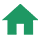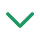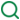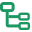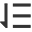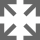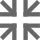## 前言

JavaScript中有一个被称为作用域(Scope)的特性。虽然对于许多新手开发者来说，作用域的概念并不是很容易理解，本文我会尽我所能用最简单的方式来解释作用域和作用域链，希望大家有所收获！

## 作用域(Scope)

### 1.什么是作用域

``````function outFun2() {
var inVariable = "内层变量2";
}
outFun2();//要先执行这个函数，否则根本不知道里面是啥
console.log(inVariable); // Uncaught ReferenceError: inVariable is not defined
``````

ES6 之前 JavaScript 没有块级作用域,只有全局作用域和函数作用域。ES6的到来，为我们提供了‘块级作用域’,可通过新增命令let和const来体现。

### 2.全局作用域和函数作用域

• 最外层函数 和在最外层函数外面定义的变量拥有全局作用域
``````var outVariable = "我是最外层变量"; //最外层变量
function outFun() { //最外层函数
var inVariable = "内层变量";
function innerFun() { //内层函数
console.log(inVariable);
}
innerFun();
}
console.log(outVariable); //我是最外层变量
outFun(); //内层变量
console.log(inVariable); //inVariable is not defined
innerFun(); //innerFun is not defined
``````
• 所有末定义直接赋值的变量自动声明为拥有全局作用域
``````function outFun2() {
variable = "未定义直接赋值的变量";
var inVariable2 = "内层变量2";
}
outFun2();//要先执行这个函数，否则根本不知道里面是啥
console.log(variable); //未定义直接赋值的变量
console.log(inVariable2); //inVariable2 is not defined
``````
• 所有window对象的属性拥有全局作用域

``````// 张三写的代码中
var data = {a: 100}

// 李四写的代码中
var data = {x: true}
``````

``````function doSomething(){
var blogName="浪里行舟";
function innerSay(){
}
innerSay();
}
innerSay(); //脚本错误
``````• 泡泡1是全局作用域，有标识符foo；
• 泡泡2是作用域foo，有标识符a,bar,b；
• 泡泡3是作用域bar，仅有标识符c。

``````if (true) {
// 'if' 条件语句块不会创建一个新的作用域
var name = 'Hammad'; // name 依然在全局作用域中
}
``````

JS 的初学者经常需要花点时间才能习惯变量提升，而如果不理解这种特有行为，就可能导致 bug 。正因为如此， ES6 引入了块级作用域，让变量的生命周期更加可控。

### 3.块级作用域

1. 在一个函数内部
2. 在一个代码块（由一对花括号包裹）内部

let 声明的语法与 var 的语法一致。你基本上可以用 let 来代替 var 进行变量声明，但会将变量的作用域限制在当前代码块中。块级作用域有以下几个特点：

• 声明变量不会提升到代码块顶部

let/const 声明并不会被提升到当前代码块的顶部，因此你需要手动将 let/const 声明放置到顶部，以便让变量在整个代码块内部可用。

``````function getValue(condition) {
if (condition) {
let value = "blue";
return value;
} else {
// value 在此处不可用
return null;
}
// value 在此处不可用
}
``````
• 禁止重复声明

``````var count = 30;
let count = 40; // Uncaught SyntaxError: Identifier 'count' has already been declared
``````

``````var count = 30;
// 不会抛出错误
if (condition) {
let count = 40;
// 其他代码
}
``````
• 循环中的绑定块作用域的妙用

``````for (let i = 0; i < 10; i++) {
// ...
}
console.log(i);
// ReferenceError: i is not defined
``````

``````var a = [];
for (var i = 0; i < 10; i++) {
a[i] = function () {
console.log(i);
};
}
a(); // 10
``````

``````var a = [];
for (let i = 0; i < 10; i++) {
a[i] = function () {
console.log(i);
};
}
a(); // 6
``````

``````for (let i = 0; i < 3; i++) {
let i = 'abc';
console.log(i);
}
// abc
// abc
// abc
``````

## 作用域链

### 1.什么是自由变量

``````var a = 100
function fn() {
var b = 200
console.log(a) // 这里的a在这里就是一个自由变量
console.log(b)
}
fn()
``````

### 2.什么是作用域链

``````var a = 100
function F1() {
var b = 200
function F2() {
var c = 300
console.log(a) // 自由变量，顺作用域链向父作用域找
console.log(b) // 自由变量，顺作用域链向父作用域找
console.log(c) // 本作用域的变量
}
F2()
}
F1()
``````

### 3.关于自由变量的取值

``````var x = 10
function fn() {
console.log(x)
}
function show(f) {
var x = 20
(function() {
f() //10，而不是20
})()
}
show(fn)
``````

``````var a = 10
function fn() {
var b = 20
function bar() {
console.log(a + b) //30
}
return bar
}
var x = fn(),
b = 200
x() //bar()
``````

fn()返回的是bar函数，赋值给x。执行x()，即执行bar函数代码。取b的值时，直接在fn作用域取出。取a的值时，试图在fn作用域取，但是取不到，只能转向创建fn的那个作用域中去查找，结果找到了,所以最后的结果是30

## 作用域与执行上下文

• 词法分析
• 语法分析
• 作用域规则确定

### 执行阶段：

• 创建执行上下文
• 执行函数代码
• 垃圾回收

JavaScript解释阶段便会确定作用域规则，因此作用域在函数定义时就已经确定了，而不是在函数调用时确定，但是执行上下文是函数执行之前创建的。执行上下文最明显的就是this的指向是执行时确定的。而作用域访问的变量是编写代码的结构确定的。

## 参考文章和书籍